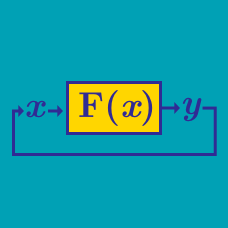Computer Science

# Functions (CS): Level 4 Challenges

The fibonacci sequence is defined as $F_0=0,F_1=1$ and for $n\geq 2$, $F_n=F_{n-1}+F_{n-2}$

Thus, the fibonacci sequence is $0,1,1,2,3,5,8,13,...$

Find the sum of all the numbers less than $\textbf{1 billion}$ which appear in the $\textbf{Fibonacci Sequence}$ and are divisible by $3$.It's your first day at work as an intern, and your boss explains a major problem: "Our only developer quit yesterday, and he left this note on his desk. We need to know what it does. If you can figure it out, we'll promote you to his job."

#PSEUDOCODE
mystery(list):
if list is empty then return the empty list
otherwise:
let list1 consist of all elements of list that are <= first element, excluding the first element itself
let list2 consist of only the first element of list
let list3 consist of all elements of list that are > first element
return mystery(list1) + list2 + mystery(list3) # + indicates list concatenation

thelist = [1, 4, 2, 3, 3, 45, 6, 7, 8, 5, 4, 3, 2, 21, 2, 3, 4, 5, 6, 7]
newlist = mystery(thelist)
total = 0
for i in range(5):
total += newlist[i]
print total


So, what is this code supposed to print?

Details and assumptions
1. To make it clear what is meant by list concatenation, here is an example: $[2, 7] + [8, 9, 3] = [2, 7, 8, 9, 3].$ 2. Note that mystery is a recursive function (it calls itself at the last step).

###### Image credit: oldcomputers.comI have an integer (in decimal representation) such that if I reverse its digits and add them up, I will get a new integer. I repeat this process until the resulting integer is a palindrome. We will denote an integer as a near-symmetric number if after twenty-five iterations, the resulting integer is still not a palindrome. What is the smallest positive near-symmetric number?

Details and assumptions

• A palindrome is a number that remains the same when its digits are reversed.

• As an explicit example, consider the integer to be $49$. The resulting number will be $49 + 94 = 143$. Repeat: $143 + 341 = 484$, which is a palindrome (after $2 < 25$ iterations). Thus $49$ is not a near-symmetric number.

×